# Class 11 Maths NCERT Solutions for Chapter 12 Introduction to Three Dimensional Geometry Exercise 12.2### Three Dimensional Geometry Exercise 12.2 Solutions

1. Find the distance between the following pairs of points:
(i) (2, 3, 5) and (4, 3, 1)
(ii) (–3, 7, 2) and (2, 4, –1)
(iii) (–1, 3, –4) and (1, –3, 4)
(iv) (2, –1, 3) and (–2, 1, 3)

Solution

The distance between points P(x1, y1, z1) and P(x2, y2, z2) is given by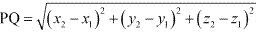(i) Distance between points (2, 3, 5) and (4, 3, 1)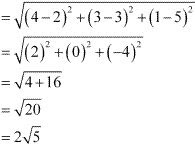(ii) Distance between points (-3, 7, 2) and (2, 4, -1)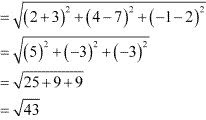(iii) Distance between points (-1, 3, -4) and (1, -3, 4)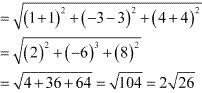(iv) Distance between points (2, -1, 3) and (-2, 1, 3)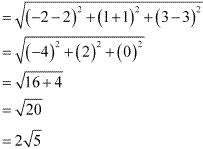2. Show that the points (–2, 3, 5), (1, 2, 3) and (7, 0, –1) are collinear.

Solution

Let points (–2, 3, 5), (1, 2, 3), and (7, 0, –1) be denoted by P, Q, and R respectively.
Points P, Q, and R are collinear if they lie on a line.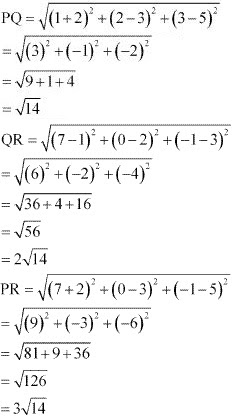Here, PQ + QR = √14 + 2√14 = 3√14 = PR
Hence, points P(-2, 3, 5), Q(1, 2, 3), and R(7, 0, -1) are collinear.

3. Verify the following:
(i) (0, 7, –10), (1, 6, –6) and (4, 9, –6) are the vertices of an isosceles triangle.
(ii) (0, 7, 10), (–1, 6, 6) and (–4, 9, 6) are the vertices of a right angled triangle.
(iii) (–1, 2, 1), (1, –2, 5), (4, –7, 8) and (2, –3, 4) are the vertices of a parallelogram.

Solution

(i) Let points (0, 7, -10), (1, 6, -6), and (4, 9, -6) be denoted by A, B, and C respectively.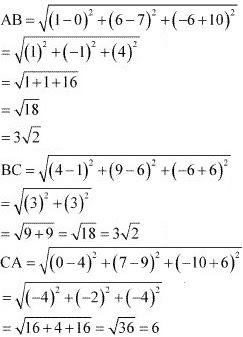Here, AB = BC ≠ CA
Thus, the given points are the vertices of an isosceles triangle.

(ii) Let (0, 7, 10), (–1, 6, 6), and (–4, 9, 6) be denoted by A, B, and C respectively.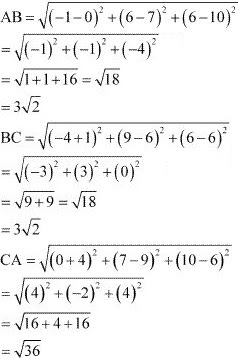Now, AB2 + BC2 = (3√2)2 + (3√2)2 = 18 + 18 = 36 = AC2.
Therefore, by Pythagoras theorem, ABC is a right triangle.
Hence, the given points are the vertices of a right - angled triangle.

(iii) Let (-1, 2, 1), (1, -2, 5), (4, -7, 8), and (2, -3, 4) be denoted by A, B, C, and D respectively.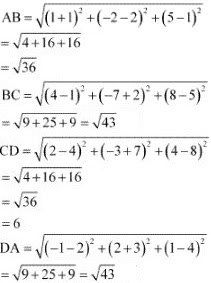Here, AB = CD = 6, BC = AD = √43
Hence, the opposite sides of quadrilateral ABCD, whose vertices are taken in order, are equal.
Therefore, ABCD is a parallelogram.
Hence, the given points are the vertices of a parallelogram.

4. Find the equation of the set of points which are equidistant from the points (1, 2, 3) and (3, 2, –1).

Solution

Let P (x, y, z) be the point that is equidistant from points A(1, 2, 3) and B(3, 2, –1).
Accordingly, PA = PB
⇒ PA2 = PB2
⇒ (x - 1)2 + (y - 2)2 + (z - 3)2 = (x -3)2 + (y - 2)2 + (z + 1)2
⇒ x2 - 2x + 1 + y2 - 4y + 4 +z2 - 6z + 9 = x2 - 6x + 9 + y2 - 4y + 4 + z2 + 2z + 1
⇒ -2x - 4y - 6z + 14 = -6x - 4y + 2z + 14
⇒ -2x - 6z + 6x - 2z = 0
⇒ 4x - 8z = 0
⇒ x - 2z = 0
Thus, the required equation is x - 2z = 0.

5. Find the equation of the set of points P, the sum of whose distances from A (4, 0, 0) and B (–4, 0, 0) is equal to 10.

Solution

Let the coordinates of P be (x, y, z).
The coordinates of points A and B are (4, 0, 0) and (–4, 0, 0) respectively.
It is given that PA + PB = 10.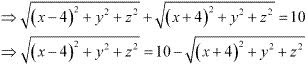On squaring both sides, we obtain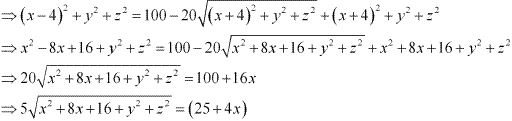On squaring both sides again, we obtain
25 (x2 + 8x + 16 + y2 + z2) = 625 + 16x2 + 200x
⇒ 25x2 + 200x + 400 + 25y2 + 25z2 = 625 + 16x2 + 200x
⇒ 9x2 + 25y2 + 25z2 – 225 = 0
Thus, the required equation is 9x2 + 25y2 + 25z2 – 225 = 0.